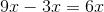# ISEE Middle Level Math : How to subtract variables

## Example Questions

### Example Question #166 : Ssat Middle Level Quantitative (Math)

Solve.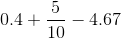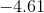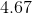None of these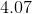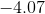Explanation:

The first step to solving is to convert all the numbers to a common form. We will convert to decimals, since this form is used in the answer choices.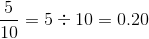Plug this value into the original equation and solve.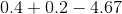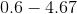Our final answer is.

### Example Question #31 : How To Subtract Variables

Ifis an odd number, which of the following must also be odd?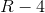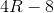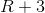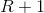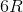Explanation:

The easiest way to solve this problem is to pick an odd number (we can use 3 for example), and plug it into each answer choice.

The only answer choice that gives us an odd result is: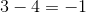As a rule of thumb, an even number subtracted from an odd number will always result in an odd number.

### Example Question #21 : How To Subtract Variables

Philip had a glass containing 16 ounces of water. He drank 2 ounces. His brother then drank half of what remained. How many ounces of water are now in the glass?Explanation:

If there were 16 ounces of water to start, this amount decreased to 14 ounces when Philip drank 2 ounces.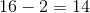With 14 ounces remaining, his brother drank half. This would be equal to 7 ounces.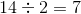This left the other half of the water, which was also 7 ounces; thus, 7 ounces in the amount of water that remained.

### Example Question #33 : How To Subtract Variables

Ifminus two times another number equals, what is the other number?Explanation:

The first step is to transferminus two times another number equalsinto mathematical terms. Doing so gives us: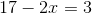Next, 17 is subtracted from each side.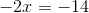Each side is now divided by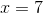Therefore,is the correct answer.

### Example Question #34 : How To Subtract Variables

Jim and Jack are having tea together. There are 10 sugar cubes on a small tray. Jim takes 1 cube for his tea, and Jack takes twice as many cubes as Jim. How many sugar cubes remain?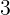Explanation:

The first step is to convert the following into a mathematical equation:

There are 10 sugar cubes on a small tray. Jim takes 1 cube for his tea, and Jack takes twice as many cubes as Jim.

The equation for the remaining cubes would be: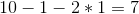Therefore, 7 is the correct answer.

### Example Question #35 : How To Subtract Variables

There are 9 cats in a shelter. Bob adopts 2 of the cats. Anita then adopts more cats than Bob adopted, but fewer than the majority of the cats remaining. How many cats did Anita adopt?Explanation:

If Anita adopted more cats than Bob, then she adopted more than 2 cats.

Given that Bob adopted 2 cats, there are 7 cats remaining. A majority of the remaining cats would be 4.

Given that Anita adopted more than 2 cats, but less than 4, she must have adopted 3 cats; therefore, 3 is the correct answer.

### Example Question #36 : How To Subtract Variables

Tom has 8 pretzel sticks. He gives one stick to his sister, and then breaks what remains in half. Tom eats 5 of the half-pretzel sticks. How many half-pretzel sticks remain?Explanation:

If Tom has 8 pretzel sticks and gives one stick to his sister, then he will have 7 pretzel sticks remaining. If he then breaks what remains in half, he will have 14 half-pretzel sticks. If Tom eats 5 of the half-pretzel sticks, 9 half-pretzel sticks will therefore remain.

### Example Question #37 : How To Subtract Variables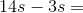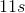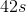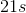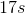Explanation:

Subtract the numbers and keep the variable: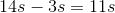Answer:### Example Question #21 : How To Subtract Variables

Simplify the following expression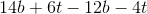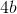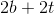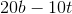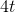Explanation:

Simplify the following expressionWe can begin by grouping like terms: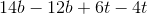Next, combine the like terms. In this case, simply treat them like two subtraction problems.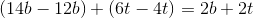### Example Question #22 : How To Subtract Variables

Simplify the following: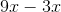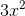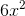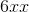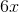Explanation:

When subtracting variables, you must first make sure you have the same variables.  So, in this casewe can see that both terms contain the variable x.  Therefore, we can subtract them.

Now, when we subtract them, we can think of the variables as objects.  So, we can say that the variable x is actually pieces of pizza.  So,is saying we have 9 pieces of pizza and we eat 3 pieces of pizza.  So how many pieces of pizza do we have left?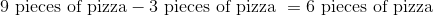We can simplify our problem the same way.